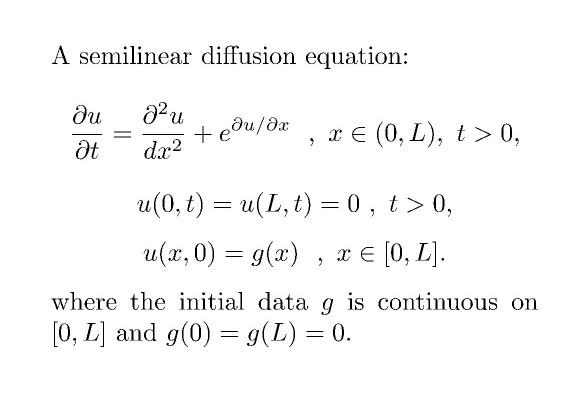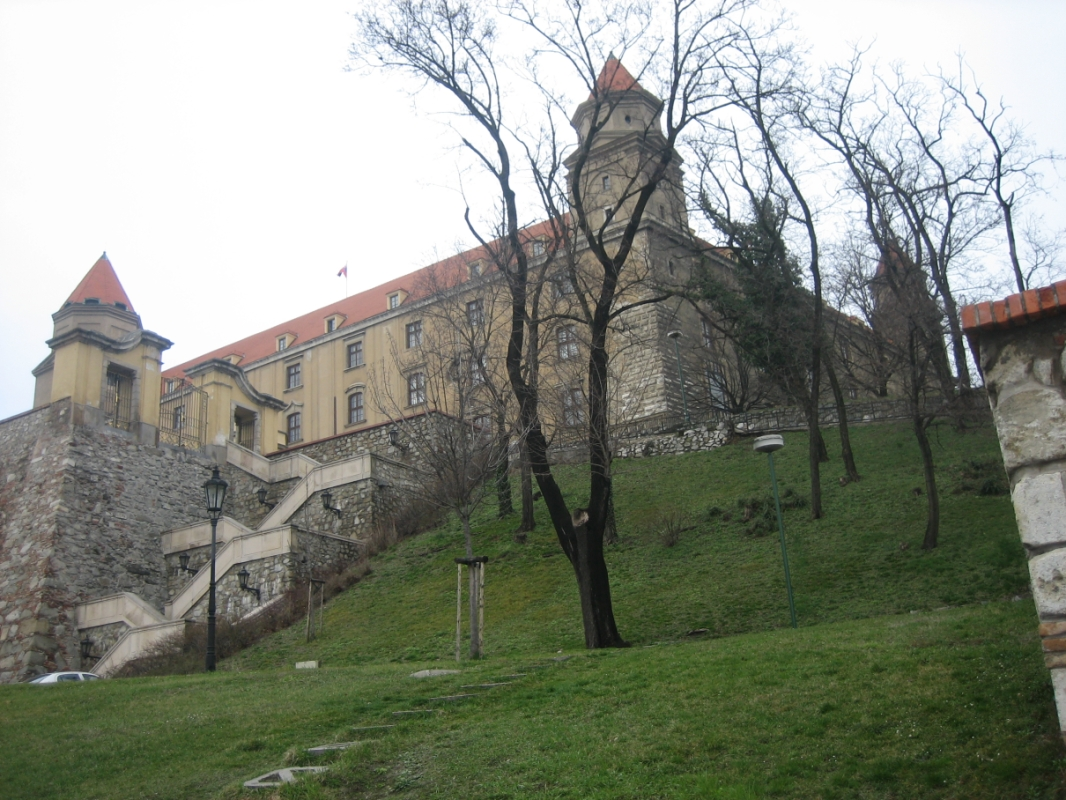Main page Department of Mathematics and Statistics of University of HelsinkiFormula 1. A semilinear equation with gradient blow-up in finite time.Figure 1. The Bratislava Castle. This research project started during the FMS International Visitor Program "Nonlinear parabolic problems" in Autumn 2005. SOME RECENT ARTICLES M.Fila, J.Taskinen, M.Winkler: Convergence to a singular steady state of a parabolic equation with gradient blow-up. Appl.Math. Letters. 20 (2007), 578-582. It is known (from a paper by Ph.Souplet and J.L.Vazquez, 2006) that the semilinear initial-boundary problem presented in Formula 1 has a bounded solution u, global in time, which however ceases to be a global classical solution: there exists a T, 0 < T < ∞ , such that first derivative u', or gradient, of u blows up at the time T. The blow-up occurs spatially at one end of the interval. On the other hand the equation has a singular steady state v(x) = -x ln x + x + C. Generalizing the solution u for times t > T, a result from the above citation shows that u → v as t → ∞. In this paper we determine the rate of this convergence: we show that || u -v || ≤ exp( - (λ - ε) t ) where λ is the expect convergence exponent coming from a linearization of the problem. (It comes from the first zero of the 0th Bessel function.) Back to main page .